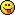## Discuss Scratch

### Calculating surface normal

I am creating a
`bounce off [Sprite1 v] :: motion`
block in BYOB, and I would like to calculate the surface normal of the sprite within the block definition, where the collision is going to take place. Using
`([direction v] of [Sprite1 v])`
works, but only for lines that are vertical in the paint editor. I've never seen a problem like this on any other website, so this might be tricky, or even impossible, to solve. Does anyone have a solution? If so, please post it here.

Last edited by rdococ (April 9, 2015 00:17:26)

### Calculating surface normal

Hmm surface normal? Well if you have a defined polygon or curve, it's easy, but are you asking how to do it for any arbitrary raster image?

```\$(".box-head").textContent = "committing AT crimes since \$whenever"
```

### Calculating surface normal

MegaApuTurkUltra wrote:

Hmm surface normal? Well if you have a defined polygon or curve, it's easy, but are you asking how to do it for any arbitrary raster image?
Yes, in a certain part where the collision must take place.

### Calculating surface normal

rdococ wrote:

MegaApuTurkUltra wrote:

Hmm surface normal? Well if you have a defined polygon or curve, it's easy, but are you asking how to do it for any arbitrary raster image?
Yes, in a certain part where the collision must take place.
You could attempt to vectorize the image and then detect the normal based on the line / curve there

```\$(".box-head").textContent = "committing AT crimes since \$whenever"
```

### Calculating surface normal

MegaApuTurkUltra wrote:

rdococ wrote:

MegaApuTurkUltra wrote:

Hmm surface normal? Well if you have a defined polygon or curve, it's easy, but are you asking how to do it for any arbitrary raster image?
Yes, in a certain part where the collision must take place.
You could attempt to vectorize the image and then detect the normal based on the line / curve there
That sounds hard to do in something like BYOB. Do you know of a fast way to do this?

### Calculating surface normal

rdococ wrote:

MegaApuTurkUltra wrote:

rdococ wrote:

MegaApuTurkUltra wrote:

Hmm surface normal? Well if you have a defined polygon or curve, it's easy, but are you asking how to do it for any arbitrary raster image?
Yes, in a certain part where the collision must take place.
You could attempt to vectorize the image and then detect the normal based on the line / curve there
That sounds hard to do in something like BYOB. Do you know of a fast way to do this?
No. You should just only support vector for collision (no embedded bitmaps either), and use the paths to calculate normals.

```\$(".box-head").textContent = "committing AT crimes since \$whenever"
```

### Calculating surface normal

MegaApuTurkUltra wrote:

rdococ wrote:

MegaApuTurkUltra wrote:

Hmm surface normal? Well if you have a defined polygon or curve, it's easy, but are you asking how to do it for any arbitrary raster image?
Yes, in a certain part where the collision must take place.
You could attempt to vectorize the image and then detect the normal based on the line / curve there

No wow that's waaaay complicated for Scratch.Here's what you do:

Move on X axis. On collision with wall, back out X so that the ball is no longer collided. Then move back 3 pixels into the wall. After that, move on the Y axis to see how many pixels it takes to get back out of the wall. If it takes too many pixels to get back out of the wall, then it's probably a vertical wall.

Move on Y axis. On collision with wall, back out Y so that the ball is no longer collided. Then move back 3 pixels into the wall. After that, move on the X axis to see how many pixels it takes to get back out of the wall. If it takes too many pixels to get back out of the wall, then it's probably a horizontal wall.

With the slopes of the lines calculated by pixel collisions, the proper velocity vector can be calculated. Here's an unfinished link to a demo thingy: https://scratch.mit.edu/projects/36255524/

### Calculating surface normal

rdococ wrote:

I am creating a
`bounce off [Sprite1 v] :: motion`
block in BYOB, and I would like to calculate the surface normal of the sprite within the block definition, where the collision is going to take place. Using
`([direction v] of [Sprite1 v])`
works, but only for lines that are vertical in the paint editor. I've never seen a problem like this on any other website, so this might be tricky, or even impossible, to solve. Does anyone have a solution? If so, please post it here.
You could move the sprite +/-1 from the initial position and the +/-2, etc., and test at each offset for touching. This would generate a boundary of touching, to which you could then attempt to fit a line and subsequently determine a normal to that line. But with a line that is only a few pixels long, it would be a very approximate solution.

G